``` [all packages] [package java.util] [class hierarchy] [index] ```

# public class java.util.Vector

(source file: Vector.java)
```java.lang.Object
|
+----java.util.AbstractCollection<A>
|
+----java.util.AbstractList<A>
|
+----java.util.Vector
```

The pure class interface.
```public class Vector
extends AbstractList
implements List<A>, Cloneable, java.io.Serializable
```
The `Vector` class implements a growable array of objects. Like an array, it contains components that can be accessed using an integer index. However, the size of a `Vector` can grow or shrink as needed to accommodate adding and removing items after the `Vector` has been created.

Each vector tries to optimize storage management by maintaining a `capacity` and a `capacityIncrement`. The `capacity` is always at least as large as the vector size; it is usually larger because as components are added to the vector, the vector's storage increases in chunks the size of `capacityIncrement`. An application can increase the capacity of a vector before inserting a large number of components; this reduces the amount of incremental reallocation.

As of JDK1.2, this class has been retrofitted to implement List, so that it becomes a part of Java's collection framework. Unlike the new collection implementations, Vector is synchronized.

The Iterators returned by Vector's iterator and listIterator methods are fail-fast: if the Vector is structurally modified at any time after the Iterator is created, in any way except through the Iterator's own remove or add methods, the Iterator will throw a ConcurrentModificationException. Thus, in the face of concurrent modification, the Iterator fails quickly and cleanly, rather than risking arbitrary, non-deterministic behavior at an undetermined time in the future. The Enumerations returned by Vector's elements method are not fail-fast.

##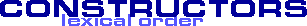Vector()
Constructs an empty vector so that its internal data array has size 10 and itsVector(Collection<A>)
Constructs a vector containing the elements of the specified collection, in the order theyVector(int)
Constructs an empty vector with the specified initial capacity and with its capacityVector(int, int)
Constructs an empty vector with the specified initial capacity and capacity increment

##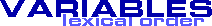capacityIncrement
The amount by which the capacity of the vector is automatically incremented when its sizeelementCount
The number of valid components in this Vector objectelementData
The array buffer into which the components of the vector are stored

##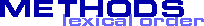add(int, Object)
Inserts the specified element at the specified position in this Vector. Shifts the elementaddAll(int, Collection<A>)
Inserts all of the elements in in the specified Collection into this Vector at theaddAll(Collection<A>)
Appends all of the elements in the specified Collection to the end of this this Vector, inaddElement(Object)
Adds the specified component to the end of this vector, increasing its size by onecapacity()
Returns the current capacity of this vector.clear()
Removes all of the elements from this Vectorclone()
Returns a clone of this vectorcontains(Object)
Tests if the specified object is a component in this vector.copyInto(Object[])
Copies the components of this vector into the specified arrayelementAt(int)
Returns the component at the specified index.elements()
Returns an enumeration of the components of this vectorensureCapacity(int)
Increases the capacity of this vector, if necessary, to ensure that it can hold at leastfirstElement()
Returns the first component (the item at index 0) of this vector.get(int)
Returns the element at the specified position in this Vector.indexOf(Object, int)
Searches for the first occurence of the given argument, beginning the search atindexOf(Object)
Searches for the first occurence of the given argument, testing for equality using theinsertElementAt(Object, int)
Inserts the specified object as a component in this vector at the specifiedisEmpty()
Tests if this vector has no components.lastElement()
Returns the last component of the vector.lastIndexOf(Object, int)
Searches backwards for the specified object, starting from the specified index, andlastIndexOf(Object)
Returns the index of the last occurrence of the specified object in this vector.remove(int)
Removes the element at the specified position in this Vector. shifts any subsequentremoveAllElements()
Removes all components from this vector and sets its size to zero.removeElement(Object)
Removes the first (lowest-indexed) occurrence of the argument from this vectorremoveElementAt(int)
Deletes the component at the specified indexremoveRange(int, int)
Removes from this Vector all of the elements whose index is between fromIndex, inclusiveset(int, Object)
Replaces the element at the specified position in this Vector with the specified element.setElementAt(Object, int)
Sets the component at the specified `index` of this vector to be the specifiedsetSize(int)
Sets the size of this vectorsize()
Returns the number of components in this vector.toArray()
Returns an array containing all of the elements in this Vector in the correct order.trimToSize()
Trims the capacity of this vector to be the vector's current size

##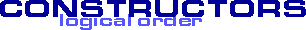Vector
```public Vector(int initialCapacity,
int capacityIncrement);
```
Constructs an empty vector with the specified initial capacity and capacity increment.

Parameters:
initialCapacity - the initial capacity of the vector.
capacityIncrement - the amount by which the capacity is increased when the vector overflows.
Throws:
IllegalArgumentException -if the specified initial capacity is negativeVector

```public Vector(int initialCapacity);
```
Constructs an empty vector with the specified initial capacity and with its capacity increment equal to zero.

Parameters:
initialCapacity - the initial capacity of the vector.
Throws:
IllegalArgumentException -if the specified initial capacity is negativeVector

```public Vector();
```
Constructs an empty vector so that its internal data array has size 10 and its standard capacity increment is zero.Vector

```public Vector(Collection<A> c);
```
Constructs a vector containing the elements of the specified collection, in the order they are returned by the collection's iterator.

##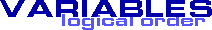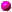elementData
```protected Object[] elementData;
```
The array buffer into which the components of the vector are stored. The capacity of the vector is the length of this array buffer, and is at least large enough to contain all the vector's elements.elementCount

```protected int elementCount;
```
The number of valid components in this Vector object. Components elementData through elementData[elementCount-1] are the actual items.capacityIncrement

```protected int capacityIncrement;
```
The amount by which the capacity of the vector is automatically incremented when its size becomes greater than its capacity. If the capacity increment is `0`, the capacity of the vector is doubled each time it needs to grow.

##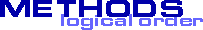copyInto
```public synchronized void copyInto(Object[] anArray);
```
Copies the components of this vector into the specified array. The item at index k in this vector is copied into component k of anArray. The array must be big enough to hold all the objects in this vector, else an IndexOutOfBoundsException is thrown.

Parameters:
anArray - the array into which the components get copied.trimToSize

```public synchronized void trimToSize();
```
Trims the capacity of this vector to be the vector's current size. If the capacity of this cector is larger than its current size, then the capacity is changed to equal the size by replacing its internal data array, kept in the field elementData, with a smaller one. An application can use this operation to minimize the storage of a vector.ensureCapacity

```public synchronized void ensureCapacity(int minCapacity);
```
Increases the capacity of this vector, if necessary, to ensure that it can hold at least the number of components specified by the minimum capacity argument.

If the current capacity of this vector is less than minCapacity, then its capacity is increased by replacing its internal data array, kept in the field elementData, with a larger one. The size of the new data array will be the old size plus capacityIncrement, unless the value of capacityIncrement is nonpositive, in which case the new capacity will be twice the old capacity; but if this new size is still smaller than minCapacity, then the new capacity will be minCapacity.

Parameters:
minCapacity - the desired minimum capacity.setSize

```public synchronized void setSize(int newSize);
```
Sets the size of this vector. If the new size is greater than the current size, new `null` items are added to the end of the vector. If the new size is less than the current size, all components at index `newSize` and greater are discarded.

Parameters:
newSize - the new size of this vector.capacity

```public int capacity();
```
Returns the current capacity of this vector.

Returns:
the current capacity (the length of its internal data arary, kept in the field elementData of this vector.size

```public int size();
```
Returns the number of components in this vector.

Returns:
the number of components in this vector.
Overrides:
size in class AbstractCollectionisEmpty

```public boolean isEmpty();
```
Tests if this vector has no components.

Returns:
`true` if and only if this vector has no components, that is, its size is zero; `false` otherwise.
Overrides:
isEmpty in class AbstractCollectionelements

```public synchronized Enumeration elements();
```
Returns an enumeration of the components of this vector. The returned Enumeration object will generate all items in this vector. The first item generated is the item at index 0, then the item at index 1, and so on.

Returns:
an enumeration of the components of this vector.
Enumeration, Iteratorcontains

```public boolean contains(Object elem);
```
Tests if the specified object is a component in this vector.

Parameters:
elem - an object.
Returns:
`true` if and only if the specified object is the same as a component in this vector, as determined by the equals method; `false` otherwise.indexOf

```public int indexOf(Object elem);
```
Searches for the first occurence of the given argument, testing for equality using the `equals` method.

Parameters:
elem - an object.
Returns:
the index of the first occurrence of the argument in this vector, that is, the smallest value k such that elem.equals(elementData[k]) is true; returns `-1` if the object is not found.
equals(Object)indexOf

```public synchronized int indexOf(Object elem,
int index);
```
Searches for the first occurence of the given argument, beginning the search at `index`, and testing for equality using the `equals` method.

Parameters:
elem - an object.
index - the index to start searching from.
Returns:
the index of the first occurrence of the object argument in this vector at position `index` or later in the vector, that is, the smallest value k such that elem.equals(elementData[k]) && (k >= index) is true; returns `-1` if the object is not found.
equals(Object)lastIndexOf

```public int lastIndexOf(Object elem);
```
Returns the index of the last occurrence of the specified object in this vector.

Parameters:
elem - the desired component.
Returns:
the index of the last occurrence of the specified object in this vector, that is, the largest value k such that elem.equals(elementData[k]) is true; returns `-1` if the object is not found.lastIndexOf

```public synchronized int lastIndexOf(Object elem,
int index);
```
Searches backwards for the specified object, starting from the specified index, and returns an index to it.

Parameters:
elem - the desired component.
index - the index to start searching from.
Returns:
the index of the last occurrence of the specified object in this vector at position less than `index` in the vector, that is, the largest value k such that elem.equals(elementData[k]) && (k <= index) is true; `-1` if the object is not found.elementAt

```public synchronized Object elementAt(int index);
```
Returns the component at the specified index.

This method is identical in functionality to the get method (which is part of the List interface).

Parameters:
index - an index into this vector.
Returns:
the component at the specified index.
Throws:
ArrayIndexOutOfBoundsException - if the index is negative or not less than the current size of this Vector object. given.
get(int), ListfirstElement

```public synchronized Object firstElement();
```
Returns the first component (the item at index 0) of this vector.

Returns:
the first component of this vector.
Throws:
NoSuchElementException - if this vector has no components.lastElement

```public synchronized Object lastElement();
```
Returns the last component of the vector.

Returns:
the last component of the vector, i.e., the component at index `size() - 1`.
Throws:
NoSuchElementException - if this vector is empty.setElementAt

```public synchronized void setElementAt(Object obj,
int index);
```
Sets the component at the specified `index` of this vector to be the specified object. The previous component at that position is discarded.

The index must be a value greater than or equal to `0` and less than the current size of the vector.

This method is identical in functionality to the set method (which is part of the List interface). Note that the set method reverses the order of the parameters, to more closely match array usage. Note also that the set method returns the old value that was stored at the specified position.

Parameters:
obj - what the component is to be set to.
index - the specified index.
Throws:
ArrayIndexOutOfBoundsException - if the index was invalid.
size(), List, setremoveElementAt

```public synchronized void removeElementAt(int index);
```
Deletes the component at the specified index. Each component in this vector with an index greater or equal to the specified `index` is shifted downward to have an index one smaller than the value it had previously. The size of this vector is decreased by 1.

The index must be a value greater than or equal to `0` and less than the current size of the vector.

This method is identical in functionality to the remove method (which is part of the List interface). Note that the remove method returns the old value that was stored at the specified position.

Parameters:
index - the index of the object to remove.
Throws:
ArrayIndexOutOfBoundsException - if the index was invalid.
size(), remove(int), ListinsertElementAt

```public synchronized void insertElementAt(Object obj,
int index);
```
Inserts the specified object as a component in this vector at the specified `index`. Each component in this vector with an index greater or equal to the specified `index` is shifted upward to have an index one greater than the value it had previously.

The index must be a value greater than or equal to `0` and less than or equal to the current size of the vector.

This method is identical in functionality to the add(Object, int) method (which is part of the List interface). Note that the add method reverses the order of the parameters, to more closely match array usage.

Parameters:
obj - the component to insert.
index - where to insert the new component.
Throws:
ArrayIndexOutOfBoundsException - if the index was invalid.addElement

```public synchronized void addElement(Object obj);
```
Adds the specified component to the end of this vector, increasing its size by one. The capacity of this vector is increased if its size becomes greater than its capacity.

This method is identical in functionality to the add(Object) method (which is part of the List interface).

Parameters:
obj - the component to be added.removeElement

```public synchronized boolean removeElement(Object obj);
```
Removes the first (lowest-indexed) occurrence of the argument from this vector. If the object is found in this vector, each component in the vector with an index greater or equal to the object's index is shifted downward to have an index one smaller than the value it had previously.

This method is identical in functionality to the remove(Object) method (which is part of the List interface).

Parameters:
obj - the component to be removed.
Returns:
`true` if the argument was a component of this vector; `false` otherwise.
remove(Object), ListremoveAllElements

```public synchronized void removeAllElements();
```
Removes all components from this vector and sets its size to zero.

This method is identical in functionality to the clear method (which is part of the List interface).

clear, Listclone

```public synchronized Object clone();
```
Returns a clone of this vector. The copy will contain a reference to a clone of the internal data array, not a reference to the original internal data array of this Vector object.

Returns:
a clone of this vector.
Overrides:
clone in class ObjecttoArray

```public synchronized Object[] toArray();
```
Returns an array containing all of the elements in this Vector in the correct order.

Overrides:
toArray in class AbstractCollectionget

```public synchronized Object get(int index);
```
Returns the element at the specified position in this Vector.

Parameters:
index - index of element to return.
Throws:
ArrayIndexOutOfBoundsException -index is out of range (index < 0 || index >= size()).
Overrides:
get in class AbstractListset

```public synchronized Object set(int index,
Object element);
```
Replaces the element at the specified position in this Vector with the specified element.

Parameters:
index - index of element to replace.
element - element to be stored at the specified position.
Returns:
the element previously at the specified position.
Throws:
ArrayIndexOutOfBoundsException -index out of range (index < 0 || index >= size()).
IllegalArgumentException -fromIndex > toIndex.add

```public synchronized void add(int index,
Object element);
```
Inserts the specified element at the specified position in this Vector. Shifts the element currently at that position (if any) and any subsequent elements to the right (adds one to their indices).

Parameters:
index - index at which the specified element is to be inserted.
element - element to be inserted.
Throws:
ArrayIndexOutOfBoundsException -index is out of range (index < 0 || index > size()).remove

```public synchronized Object remove(int index);
```
Removes the element at the specified position in this Vector. shifts any subsequent elements to the left (subtracts one from their indices). Returns the element that was removed from the Vector.

Parameters:
index - the index of the element to removed.
Throws:
ArrayIndexOutOfBoundsException -index out of range (index < 0 || index >= size()).
Overrides:
remove in class AbstractListclear

```public synchronized void clear();
```
Removes all of the elements from this Vector. The Vector will be empty after this call returns (unless it throws an exception).

Throws:
UnsupportedOperationException -clear is not supported by this Set.
Overrides:
clear in class AbstractCollectionaddAll

```public synchronized boolean addAll(Collection<A> c);
```
Appends all of the elements in the specified Collection to the end of this this Vector, in the order that they are returned by the specified Collection's Iterator. The behavior of this operation is undefined if the specified Collection is modified while the operation is in progress. (This implies that the behavior of this call is undefined if the the specified Collection is this Vector, and this Vector is nonempty.)

Parameters:
index - index at which to insert first element from the specified collection.
c - elements to be inserted into this Vector.
Throws:
ArrayIndexOutOfBoundsException -index out of range (index < 0 || index > size()).removeRange

```public synchronized void removeRange(int fromIndex,
int toIndex);
```
Removes from this Vector all of the elements whose index is between fromIndex, inclusive and toIndex, exclusive. Shifts any succeeding elements to the left (reduces their index). This call shortens the Vector by (toIndex - fromIndex) elements. (If toIndex==fromIndex, this operation has no effect.)

Parameters:
fromIndex - index of first element to be removed.
fromIndex - index after last element to be removed.
Throws:
ArrayIndexOutOfBoundsException -fromIndex or toIndex out of range (fromIndex < 0 || fromIndex >= size() || toIndex > size() || toIndex < fromIndex).
Overrides:
removeRange in class AbstractListaddAll

```public synchronized boolean addAll(int index,
Collection<A> c);
```
Inserts all of the elements in in the specified Collection into this Vector at the specified position. Shifts the element currently at that position (if any) and any subsequent elements to the right (increases their indices). The new elements will appear in the Vector in the order that they are returned by the specified Collection's iterator.

Parameters:
index - index at which to insert first element from the specified collection.
c - elements to be inserted into this Vector.
Throws:
ArrayIndexOutOfBoundsException -index out of range (index < 0 || index > size()).

``` [all packages] [package java.util] [class hierarchy] [index] ```